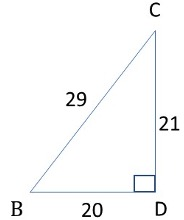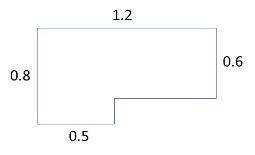Start Now with 100 Free Questions

# What does the PSAT Math Section test?

The Math section of the PSAT assesses your ability to solve problems, reason quantitatively, and manipulate mathematical expressions. The content areas covered include:

1. Heart of Algebra: This section focuses on linear equations, inequalities, and systems of equations. It tests your ability to analyze and solve problems involving linear relationships.
2. Problem Solving and Data Analysis: This area involves interpreting and analyzing data presented in various formats such as graphs, tables, and charts. It also covers ratios, percentages, proportions, and other quantitative skills needed for real-world problem-solving.
3. Advanced Math: This section includes more complex math topics like quadratic equations, polynomials, rational and radical expressions, and advanced algebraic functions. It may also cover concepts from precalculus.
4. Geometry and Trigonometry: This category can include problems that focus on area and volume; angles, triangles, and trigonometry; and circles.

The questions in the PSAT Math section vary in difficulty, ranging from easy to challenging. Some questions may require straightforward application of mathematical concepts, while others might involve multiple steps and deeper reasoning.

Keep in mind that the PSAT is often used as a practice test for the SAT and is also used for National Merit Scholarship qualification. Test content and formats can change, so TestingMom recommends checking with official sources or the College Board for the most up-to-date information about the PSAT Math section.

## PSAT Math (no calculator) Sample Questions:

Sample Question #1
Which of the following is equivalent to the expression below?

7a + 6b – 5a – (4b + 3a – 2b)

1. -a
2. -a + 4b
3. a + 4b
4. 5a + 4b

Answer: B – Combine like terms and distribute the negative: (7a – 5a – 3a) + (6b – 4b + 2b) = -a + 4b

Sample Question #2
(4 x 105)(5 x 104) is equivalent to which of the following?

1. 0.2 x 109
2. 2 x 109
3. 9 x 109
4. 2 x 1010

Answer: D – (4 x 5) x (105 x 104) = 20 x 105+4 = 20 x 109 = 2 x 1010

Sample Question #3
If a square has 3 vertices at (0, 3), (3, 6), and (3, 0), what could be the coordinates of the 4th vertex?

1. (0, 6)
2. (3, -3)
3. (6, 3)
4. (6, 6)

Answer: C – The 4th coordinate of the square is (6, 3).

Sample Question #4
If the product of 3 consecutive integers is 60, which of the following equations can be used to determine the integers?

1. x3 + 3x = 60
2. x3 + 2x2 + 2x = 60
3. x3 + 3x2 + 2x = 60
4. x3 + 3x2 + 3x = 60

Answer: C – 1st integer = x, 2nd integer = x + 1, 3rd integer = x + 2; x(x+1)(x+2) = 60,  x(x2+3x+2) = 60, x3 + 3x2 + 2x = 60

## PSAT Math (with calculator) Sample Questions:

For a list of permitted calculators on the calculator portion of the math test CLICK HERE.

Sample Question #1
Triangle BCD is shown below:What is cos B ?

1. 0.689
2. 0.724
3. 0.952
4. 1.45

Answer: A – CosB = adjacent/hypotenuse, CosB = 20/29, CosB = 0.689

Sample Question #2
Phoenix is a freelance Math Writer. If she is paid \$500 for every 50 math problems that she writes, how many math problems must she write to earn \$4900?

1. 410
2. 480
3. 490
4. 4900

Answer: C – \$500/50 = \$10 per math problem, let x = number of math problems, 10x = \$4900, x = 490; Phoenix must write 490 math problems to earn \$4900.

Sample Question #3
What is the area of the figure below, given that all angles are 90?1. 0.82 un2
2. 0.94 un2
3. 8.2 un2
4. 9.4 un2

Answer: A – Divide the figure into two rectangles and multiply their L x W. Rectangle A is 0.8 x 0.5 = 0.4.  Rectangle B is 0.6 x (1.2 – 0.5) = 0.42. Total area of the figure is Rectangle A + Rectangle B = 0.4 + 0.42 = 0.82 un2.

Sample Question #4
On Monday, Johnny bought stock in Coca-Cola for \$54/share. On Tuesday, the price increased by 5%, and on Wednesday, the price decreased by 2%. What was the stock price at the end of Wednesday?

1. \$54.57
2. \$55.57
3. \$56.57
4. \$56.70

Answer: B – Stock was purchased at \$54 per share. Tuesday the price increased 5% = \$54 x 1.05 = \$56.70, Wednesday the price decreased by 2% = \$56.70 x 0.98 = \$55.57.